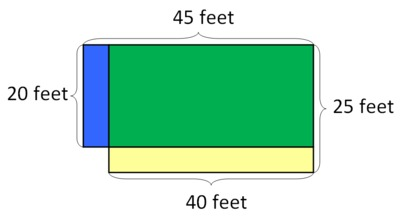# Karl's Garden

Alignments to Content Standards: 4.MD.A.3 4.OA.A.3

Karl's rectangular vegetable garden is 20 feet by 45 feet, and Makenna's is 25 feet by 40 feet. Whose garden is larger in area?

## IM Commentary

The purpose of the task is for students to solve a multi-step multiplication problem in a context that involves area. In addition, the numbers were chosen to determine if students have a common misconception related to multiplication. Since addition is both commutative and associative, we can reorder or regroup addends any way we like. So for example,

\begin{align} 20+ 45 &= 20 + (5 + 40)\\ &= (20 + 5) + 40\\ &= 25 + 40 \end{align}

Sometimes students are tempted to do something similar when multiplication is also involved; however this will get them into trouble since $$20\times (5+40) \neq (20+5) \times 40$$ This task was adapted from problem #20 on the 2011 American Mathematics Competition (AMC) 8 Test. Observers might be surprised that a task that was historically considered to be appropriate for middle school aligns to an elementary standard in the Common Core. In fact, if the factors were smaller (since in third grade students are limited to multiplication with 100; see 3.OA.3), this task would be appropriate for third grade: "3.MD.7.b Multiply side lengths to find areas of rectangles with whole-number side lengths in the context of solving real world and mathematical problems, and represent whole-number products as rectangular areas in mathematical reasoning." For example, we could use a 5 ft by 12 ft garden, and a 7 ft by 10 ft garden to make this appropriate for a (challenging) third grade task. This earlier introduction to the connection between multiplication and area brings states who have adopted the Common Core in line with other high-achieving countries. The responses to the multiple choice answers for the original problem had the following distribution:

 Choice Answer Percentage of Answers (A) Karl’s garden is larger by 100 square feet. 5.43 (B) Karl’s garden is larger by 25 square feet. 1.99 (C) The gardens are the same size. 12.75 (D) Makenna’s garden is larger by 25 square feet 2.86 (E)* Makenna’s garden is larger by 100 square feet. 76.59 Omit -- 0.37

Of the 153,485 students who participated, 72,648 or 47% were in 8th grade, 50,433 or 33% were in 7th grade, and the remainder were less than 7th grade. As the Common Core gets implemented, we will have an opportunity to compare how the generation of students who have had instructional opportunities shaped by the Common Core do on such tasks.

## Solutions

Solution: 1

We multiply the length and the width to find the area of each rectangular garden. Since $$20 \times 45 = 900$$ we have that Karl's garden is 900 square feet.

We also know that $$25 \times 40 = 1,000$$

so Makenna's garden is 1,000 square feet.

Finally, we can find the difference of the two areas

$$1,000 - 900 = 100$$

and we see that Makenna's garden is larger by 100 square feet.

Solution: With pictures

If we draw pictures to scale, we can see this difference visually. First, draw the two rectangles to represent the two gardens; the blue rectangle represents Karl's garden and the yellow rectangle represents Makenna's garden:Now, draw them overlapping. In the picture below, the green region shows where the rectangles overlap, the blue strip on the left shows the part of the blue rectangle that is not overlapped by the yellow rectangle, and the yellow strip on the bottom shows the part of the yellow rectangle that is not overlapped by the blue rectangle:Note that the blue strip is 20 feet by 5 feet and has an area of 100 square feet. The yellow strip is 40 feet by 5 feet and has an area of 200 square feet. Since $$200-100 = 100$$ we have that Makenna's garden is 100 square feet larger than Karl's garden.

If students happen to display the misconception mentioned in the commentary, then these pictures could be used to help them understand why the areas are not equal.